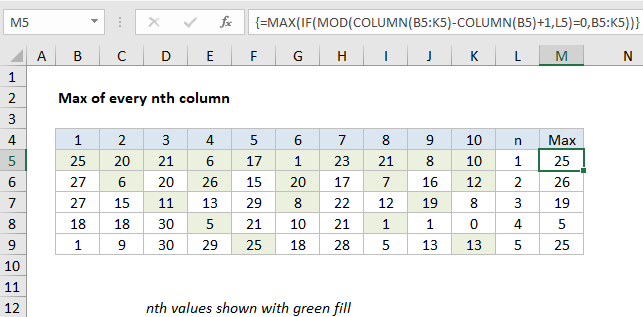## Excel Office

Excel How Tos, Tutorials, Tips & Tricks, Shortcuts

# Max of every nth column in Excel

This tutorial shows how to calculate Max of every nth column in Excel using the example below;

## Formula

`{=MAX(IF(MOD(COLUMN(range)-COLUMN(range.first)+1,n)=0,range))}`## Explanation

To get the max of every nth column, you can use an array formula based on the MAX, MOD, and COLUMN functions. In the example shown, the formula in M5 is:

`=MAX(IF(MOD(COLUMN(B5:K5)-COLUMN(B5)+1,L5)=0,B5:K5))`

Note: this is an array formula and must be entered with control + shift + enter.

### How this formula works

This formula uses the MOD and COLUMN functions to filter out values not in nth columns, then runs MAX on the result.

Worked Example:   Excel MAX, MATCH and ADDRESS function illustration

The key is this snippet:

`MOD(COLUMN(B5:K5)-COLUMN(B5)+1,L5)=0`

Here, the formula uses the COLUMN function to get a set of “relative” column numbers for the range (explained in detail here) which looks like this:

{1,2,3,4,5,6,7,8,9,10}

This array goes into the MOD function as the number argument:

`MOD({1,2,3,4,5,6,7,8,9,10},K5)=0`

where L5 is the the value to use for “nth”. The MOD function returns the remainder for each column number divided by N. So, for example, when N = 3, MOD will return an array like this:

Worked Example:   Add decimal minutes to time in Excel

{1,2,0,1,2,0,1,2,0,1}

Note that zeros appear for columns 3, 6, 9, etc., corresponding to every 3rd column. This array is compared to zero with the logical expression =0 to force a TRUE when the remainder is zero and a FALSE when not. These values go into the IF function as the logical test. The IF function filters accordingly, so only values in the original range in nth columns make it into the final array. The other values become FALSE.

Worked Example:   Extract time from a date and time in Excel

When n = 3, the final array inside MAX looks like this:

`MAX({FALSE,FALSE,11,FALSE,FALSE,8,FALSE,FALSE,19,FALSE})`

Finally, the MAX function ignores FALSE values and returns the maximum of remaining values, 19.

### Max of every other column

If you want to get the max of every other column, just adapt this formula as needed, understanding the formula automatically assigns 1 to the first column in the range. To get the max of EVEN columns, use:

`{=MAX(IF(MOD(COLUMN(A1:Z1)-COLUMN(A1)+1,2)=0,rng))}`

To get the max of ODD columns, use:

`{=MAX(IF(MOD(COLUMN(A1:Z1)-COLUMN(A1)+1,2)=1,rng))}`